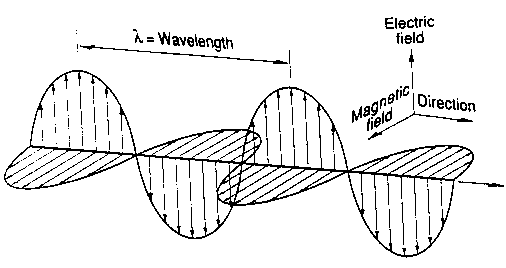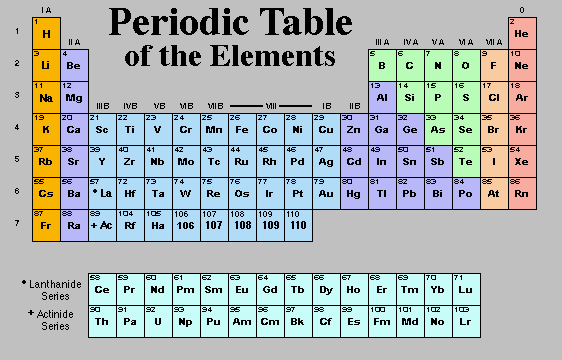## What is Astronomy? (v)

(BEING CONTINUED FROM  18/01/14)

### Lecture 7: Light, and Atoms

#### What is Light?

• Moves through a vacuum a constant speed, c.
• Exhibits Wave Nature
• Diffraction
• Exhibits Particle Nature
• Reflection

Visible light is the light that human eyes are sensitive to. It comes in colors as seen when white light is passed through a prism and broken into a rainbow.

Red Orange Yellow Green Blue Indigo Violet

Different colors correspond to electromagnetic waves having different wavelengths .

The waves consist of self-propagating, oscillating electric and magnetic fields.
Electric Field — the region in which electric forces can be observed, e.g. near an electric charge. As a field, it may also be viewed as a region of space modified by the presence of electric charges.
Magnetic field — a region in which magnetic forces can be observed. Magnetic fields are created by moving charges (current).

Changing electric fields create magnetic fields, and changing magnetic fields induce changing electric fields. If a charge accelerates the electric field around it will change, creating a magnetic field, which as it forms and collapses with induce an electric field, and an electromagnetic wave is born…Properties of waves
Wavelenth =(greek “lambda”) = distance from one crest to another. Typical units forare angstroms, 1 Å = 10-10 m = 0.1 nanometers (nm).

Frequency =(Greek “nu”) = number of times per unit time a crest passes some fixed point (say the sunflower in the above picture). Typical unit for frequency is hertz, 1 Hz = 1 s-1. [NOTE:-1 = 1/= P = Period = length of time between the passing of one crest to another as seen from a fixed point]

Speed: the speed, v, of a wave is the distance that a crest travels per unit time. We can write that in terms of wavelength and frequency as= v = c

Where, c, is the specific value that we use for the speed of electromagnetic waves. It is a constant in empty space (a vacuum).

c = 3 x 108 m/s

Wavelengths of the visible colors:

White Light: Is the presence of all the colors with equal intensities. If, for example, we had the presence of all the colors but red was more intense than the others then the light would look reddish-white, or pinkish.

#### The Electromagnetic Spectrum

Visible light is only a very tiny fraction of the total amount of possible wavelengths that an electromagnetic wave can take on. The entire range of wavelengths (or equivalently, frequencies) is referred to as the  electromagnetic spectrum.

There are no qualitative differences in these forms of radiation, but we must use very different techniques to detect them. The human eye only sees a tiny fraction of the entire spectrum.

#### Energy Carried by Electromagnetic radiation

Light can behave as discrete particles: Photons
Photons are little “wave packets” of energy

Collectively, lots of photons having the same color () make up an electromagnetic wave, with wavelength. White light is then made up of equal numbers of photons of all wavelengths.

Photons have energy but no mass. Quantum mechanics describes the energy, E, of a single photon with wavelength,as

E = hc/= hWhere h is known as Planck’s constant (h = 6.63 x 10-34 Joules*seconds, 1 Joule = 1 kg*m2/s2)

So shorter wavelength radiation has more energy than longer wavelength radiation. X-rays are more energetic than Microwaves.

Brightness (intensity): a measure of the amount of energy being received by an observer. Can be thought of as the number of photons (the greater the number the greater the brightness) or the amplitude of the electromagnetic waves.

A blackbody is an idealized object that absorbs all radiation that falls on it (hence the name “black”) and also re-emits all of that radiation. For this idealized object the manner in which it emits radiation isindependent of its composition. It depends only on the Temperature of the object. Most of the dense bodies that we will encounter in this class will be fairly decent approximations to a blackbody.

The Energy Spectrum is a plot of the intensity of radiation vs. wavelength. So this tells you how many photons are being emitted (or received) at each wavelength. For a blackbody the resulting curve is simply known as a “blackbody curve”. It is shown in figure 3.6 of the text.

The shape of the curve depends only on temperature. There is a simple relationship between the Temperature (measured in Kelvins) of the blackbody and the peak of its energy spectrum. It is known as Wien’s Law:peakT = 2.898 x 10-3 m*K

So this says that the wavelength (color) where the curve peaks is inversely proportional to the Temperature. As the temperature increases the peak moves to shorter wavelengths of radiation. And so we see the color of the object change as it is heated or cools. When you turn on the burner of an electric oven it starts out glowing a red color and as it heats up goes from red to orange to yellow. The burner is emitting radiation even before you first see it glow red. It starts out emitting IR radiation because it’s temperature is initially around 300 Kpeak = 2.898 x 10-3 m*K/(300 K) = 9.7 x 10-6 m

#### Atoms and Spectra

Structure of Atoms
Atoms are made up of 3 subatomic particles:

• e, electron: negatively charged, fundamental particle, size not measured, located in a cloud around the nucleus with size ~ 10-10 m
• p+, proton: positively charged, made up of quarks, size ~ 10-15 m, located in the nucleus
• n, neutron: electrically neutral, made up of quarks, size ~ 10-15 m, located in the nucleus

mp/me = 1840 and mp  mn

Atoms have equal numbers of protons and electrons and are electrically neutral overall.

Ions have an imbalance in protons and electrons and are electrically charged.

The chemical Elements correspond to atoms with different numbers of protons. For example, 1 proton -> Hydrogen, 2 protons -> Helium, 6 protons -> Carbon, etc.*The electrons can only occupy discrete energy levels*

In order for an electron to make a transition from one level to the other it must change its energy by an amount equal to the difference in the levels.

When an electron drops from a higher level to a lower level it must conserve energy. It must give up some energy, the amount being equal to the difference in the two energy levels. How does it do that? Electromagnetic radiation. The electron accelerates during the transition, which creates an electromagnetic wave as described earlier. The wave is a photon, whose energy is exactly equal to the difference in the energy levels, E = hc/. And so the wavelength (color) of the light emitted is dependent on how far the electron fell (how much energy it lost in the transition). The more the energy difference the shorter (bluer) the wavelength. This process is called Emission

An electron can jump up to a higher level if it can absorb a photon with just the exact energy (ie wavelength) equal to the difference in the two energy states. This process is called Absorption

Spectra

The process of absorption and emission produce rather distinctive spectra.

If you are looking at a diffuse gas of helium with a device that spreads light into its constituent colors (i.e. a prism, called a spectrograph) you will see colored lines rather than a full rainbow. These lines correspond to the wavelengths of the photons emitted by the atoms in the gas as the electrons fall from higher (excited) energy states to lower states in the helium atoms. Because the electron can only occupy specific and finite energy states there can only be a finite and specific number of lines produced by the gas. This is known as an Emission Spectrum.

Every single, atom, ion, isotope, and element has a unique pattern of emission spectrum — It’s a finger print for elements! So we can identify which elements are present in such a gas by the emission lines it produces.

A couple of things can cause an electron to be in an excited state: collisions with other atoms or absorption of photons. Think again of the blackbody. It’s an object that absorbs all radiation that falls on it. That means that no matter what elements make up the blackbody there are essentially no photons that don’t have just the right energy to excite an electron somewhere in the blackbody. Now as the blackbody absorbs energy the atoms within jiggle about and collide with one another. This can cause electrons to jump up to excited states as well. It can also cause them to fall back down and hence emit a photon. Electrons may also spontaneously fall down and emit photons. So with so many electrons absorbing and emitting the spectrum of a blackbody appears continuous. All the individual lines have run together. Thus, when you look at the emission from a blackbody with a spectrograph you will see a continuous rainbow of colors. This is called a Continuous Spectrum.

If you view the light of a blackbody’s continuous spectrum after it has passed through a diffuse and cold gas cloud something interesting will happen. The atoms in the gas cloud will absorb certain wavelengths of light corresponding to the energy levels within the atoms. The continuous spectrum when viewed through a spectrograph will then look like the rainbow with dark lines eaten out of it where the gas cloud has absorbed photons. This is called an Absorption Spectrum. We can use absorption spectra to figure out what elements are in the intervening gas cloud.

(TO BE CONTINUED)